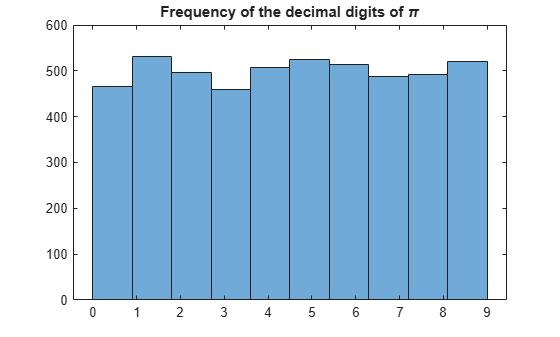# Decimal Digits of PI

This example shows how to use variable-precision arithmetic to investigate the decimal digits of pi using Symbolic Math Toolbox™.

$\pi \approx 3.1415926535897932384626433832795028841971693993751058209749445923078164062862089986280348...$

Joke: What do you get when you take the sun and divide its circumference by its diameter?

Answer: $\pi$ in the sky!

It is an old game to search for one's birthday or telephone number in the decimal digits of $\pi$. The precision of the built-in datatypes suffices to obtain a few digits only:

num2str(pi, 100000)
ans = '3.141592653589793115997963468544185161590576171875' 

The function vpa uses variable-precision to convert symbolic expressions into symbolic floating-point numbers. Convert pi to a floating-point number using vpa. Increase the precision of vpa using digits.

digits(5000); a = vpa(pi)
a =

To convert a variable-precision number into a string, use the function char.

c = char(a);

Search for your phone number in the digits of pi.

strfind(c, '1185480')
ans = 447 

It is common belief that all digits occur asymptotically equally often in the decimal expansion of $\pi$, but no proof exists yet. Find the decimal point:

pos = strfind(c, '.')
pos = 2 

Convert the decimal digits to numbers, and plot a histogram of their frequency:

d = arrayfun(@str2num, c(pos+1:end)); histogram(d, 10); title('Frequency of the decimal digits of \pi');